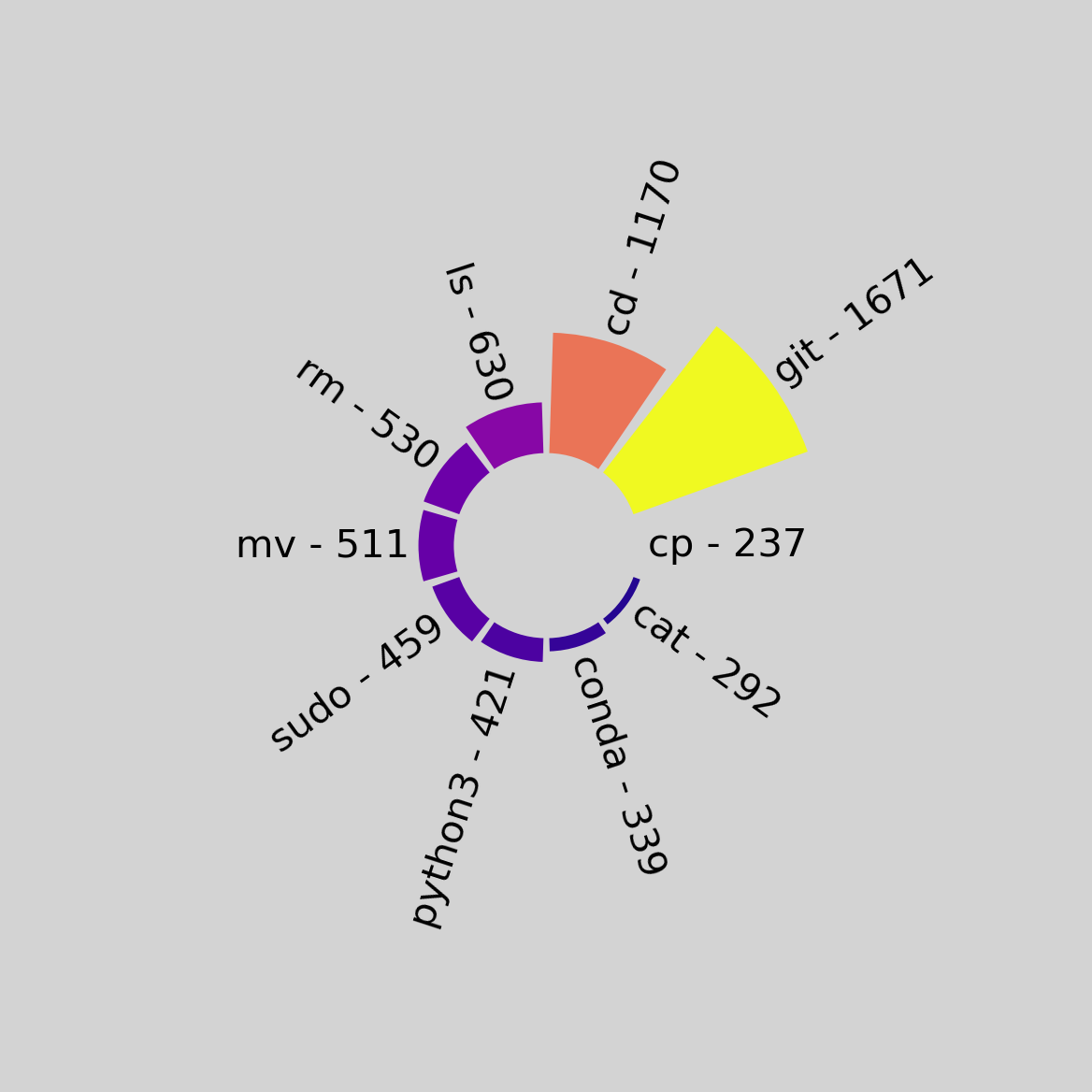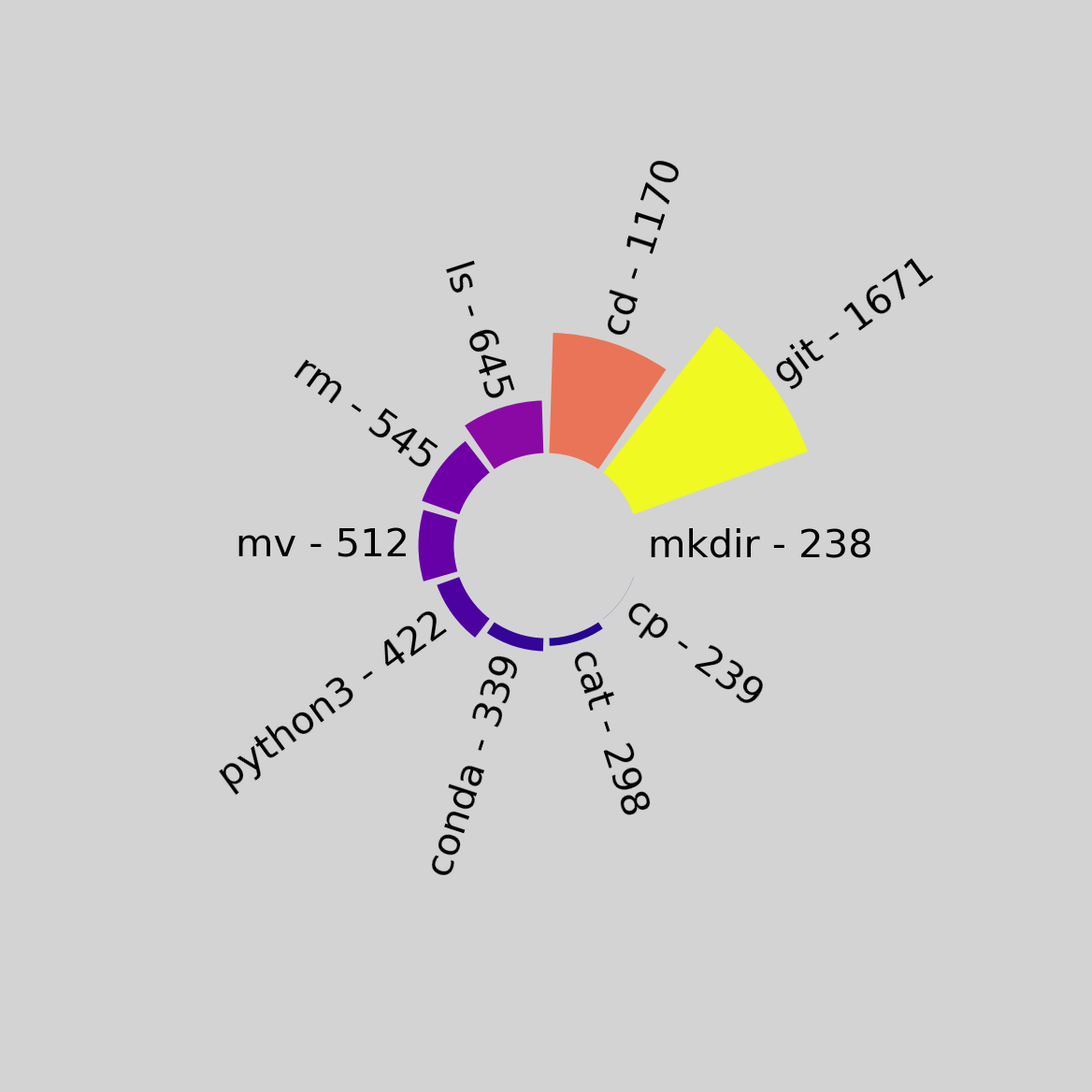When you spend enough time on the command line, you start to notice that you use certain commands a lot. Things like cd, git, and sudo get used a lot when you use Linux as your daily driver. Inspired by this Reddit post, I decided to create a tool to visualize the data.

## Streaming data

Since different shells will provide different history files, a command that parses different history files will be time-consuming to write. But most shells provide a history command that simply provides output like this:

 9989  conda activate data_analytics
9990  ipython
9991  make
9992  make clean
9993  make
9994  bat
9995  make
9996  cd ~/work
9998  ls
10000  ipython


This can be streamed to a tool with a Unix pipe:

history | some-tool


Here’s how I did this in Python:

cmds = []
while line:
matches = re.match(r"\s*[0-9]+  (.*)", line)
if not matches:
continue
parts = matches.group(1).split(" ")
cmd = parts.pop(0)


This works well enough, but in a *nix shell you can set environment variables for the duration of a command like this:

ENV_VAR=foo some-command


We don’t want to see environment variables in the output, so we need to skip those:

while re.match(r"[a-zA-Z_][a-zA-Z_0-9]*=.* ", cmd):
cmd = parts.pop(0)


At this point, I realized that sudo “hides”, in some senses, the commands that are actually being executed. When I run sudo emacs, I’m not running sudo to run sudo; I’m running Emacs with elevated privileges, which is done with the sudo command. It might be nice to be able to strip the sudo out and see the underlying command. I added argument parsing with argparse and updated the loop that finds the actual command:

while re.match(r"[a-zA-Z_][a-zA-Z_0-9]*=.* ", cmd) or (
args.strip_sudo and cmd == "sudo"
):
cmd = parts.pop(0)


The full loop is now:

while line:
matches = re.match(r"\s*[0-9]+  (.*)", line)
if not matches:
continue
parts = matches.group(1).split(" ")
cmd = parts.pop(0)
while re.match(r"[a-zA-Z_][a-zA-Z_0-9]*=.* ", cmd) or (
args.strip_sudo and cmd == "sudo"
):
cmd = parts.pop(0)
if cmd:
cmds.append(cmd)


Now we can count everything with the collections.Counter, and load the result into a pandas DataFrame:

df = pd.DataFrame(Counter(cmds).items(), columns=["command", "count"])


The number of commands to plot can be controlled with a flag:

n_largest = df.nlargest(args.n, ["count"])


Now we’re on to the plotting. Here’s the function signature:

def circle_plot(
data: np.ndarray,
labels: List[str],
max_length: int=100,
ylim_min: int=-50,
cmap: str="viridis",
background_color: str="gray",
):
""" Produce circular plot of data

Args:
data (np.ndarray): Data array to plot
labels (List[str]): String labels for data
max_length (int, optional): Maximum length of bars, defaults to 100
ylim_min (int, optional):
Minimum y-value of plot, used to tune how close the bottom of
the bars are to each other, defaults to -50
cmap (str, optional): Matplotlib colormap to use, defaults to 'viridis'
Padding between labels and the end of bars, defaults to 5
background_color (str, optional):
Background color of plot, defaults to 'gray'
"""


We start by normalizing the data:

# Normalize data
if not isinstance(data, np.ndarray):
data = np.array(data)

data_max: np.int64 = np.max(data)
data_min: np.int64 = np.min(data)
data_norm: np.ndarray = data.copy()
data_norm: np.ndarray = (data_norm - data_min) / (data_max - data_min)


Normalizing the data gives us values that are strictly in the interval [0, 1], mapping a value of 0 to 0, and the highest value to 1. This converts the visualization of each data point to be relative to the others. If there’s a command that’s used much more often than the next-closest count, we don’t want a lopsided plot with a single huge bar and lots of tiny bars.

Next, we compute the bars:

# Compute bar characteristics
bar_width: float = 2 * np.pi / len(data)
bar_angles: np.ndarray = np.arange(1, len(data) + 1) * bar_width


To plot the bars in a circle, we use a polar projection, which changes how the arguments to Axes.bar are handled, and then we plot the bars:

fig, ax = plt.subplots(subplot_kw={"projection": "polar"})

if isinstance(cmap, str):
cmap = plt.get_cmap(cmap)

bars = ax.bar(
bar_angles,
data_norm * max_length,
width=bar_width * 0.9,
color=cmap(data_norm),
zorder=10,
)


To get thing nicely scaled, a y-axis limit was empirically determined, and the ticks are removed for aesthetics:

ylim_max: float = max_length * 2.3

ax.set_ylim(ylim_min, ylim_max)
ax.set_yticks([])
ax.set_xticks([])


To plot the labels, we do some quick trigonometry and figure out where on the unit circle the bar angle will be. This lets us set the rotation and alignment.

for label, count, bar_angle, bar in zip(labels, data, bar_angles, bars):
rot = np.degrees(bar_angle)
if np.pi / 2 <= bar_angle <= 3 * np.pi / 2:
rot += 180
rot %= 360
alignment = "right"
else:
alignment = "left"
ax.text(
bar_angle,
s=f"{label} - {count}",
va="center",
rotation=rot,
rotation_mode="anchor",
ha=alignment,
)


Some additional aesthetics and returning the figure and axis:

ax.grid(False)
ax.set_facecolor(background_color)
fig.set_facecolor(background_color)
for spine in ax.spines.keys():
ax.spines[spine].set_visible(False)

return fig, ax


The outputs of the script:Running with the --strip-sudo flag:The code is available here, if you’d like to try it out yourself!